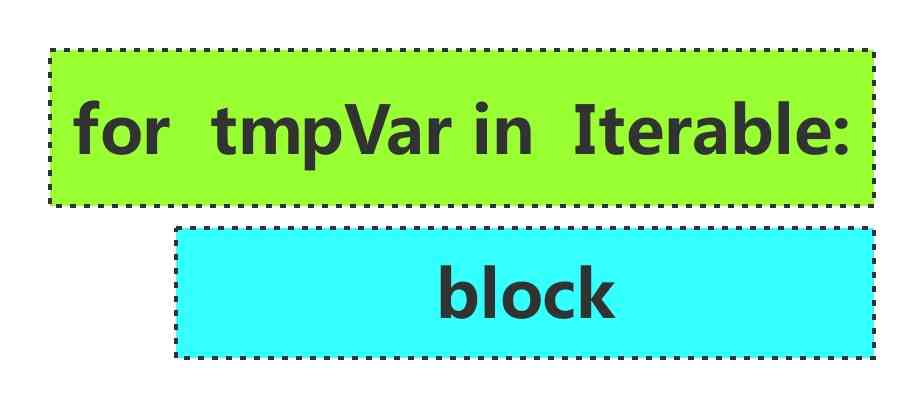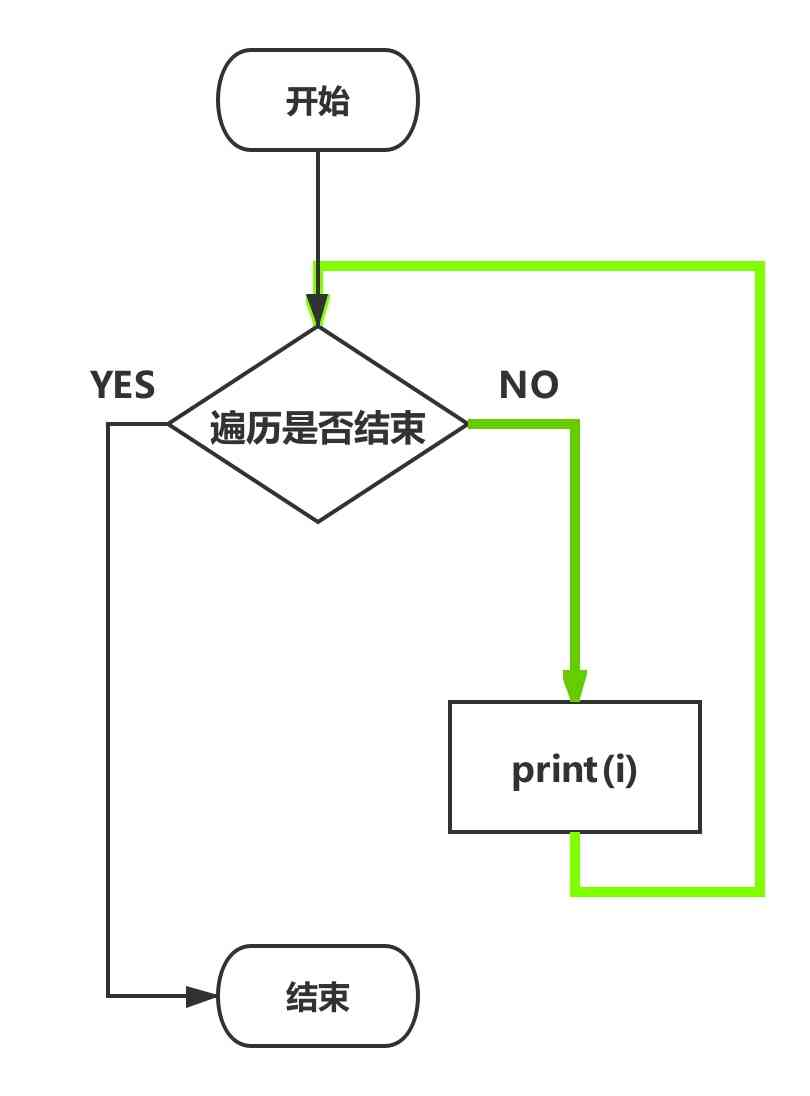# python for循环

Python for循环可以遍历任何可迭代对象，比如列表，字典，集合，字符串等，通过for循环，可以对可迭代对象里的每一个元组执行一组语句，从原理上看，python的for循环与其他编程语言的for循环不太相似，更像是其他面向对象语言中的迭代器方法1. tmpVar 是临时变量
2. Iterable 是可迭代对象
3. 绿色区域是循环条件，当对可迭代对象的迭代遍历结束时，for循环也就结束
4. block是循环体，每一次循环，程序都要进入到这个block内来执行代码

for循环，从形式上可以分为两种

1. 使用range函数自由控制循环次数
2. 遍历容器

## 1. range函数

range函数返回一个对象，该对象产生一个整数序列。

range函数有3个参数

1. start 整数序列开始的位置
2. stop 整数序列结束的位置
3. step 步长

1. range(1, 5) 产生的整数序列是1， 2， 3， 4
2. range(0, 4, 2) 产生的整数序列是0，2
3. range(5, 0, -1) 产生的整数序列是5， 4， 3， 2， 1
4. range(3) 产生的整数序列是0，1，2

## 2. 使用range函数编写for循环

``````for i in range(1, 11):
print(i)
``````

range(0, 11) 产生了整数序列:1， 2， 3， 4， 5， 6， 7， 8， 9， 10 ，结合上一篇教程《迭代遍历思维》的内容来理解这段代码。 range函数产生了一个整数序列，从0到10，for循环的过程就是迭代遍历他们的过程。第一行代码里的i是临时变量，每一次循环，都会改变i的值，i将依次等于1，2 ... 9, 10，而每一次将新的值赋值给i以后，都要进入到循环体里执行代码，在这个示例代码中，循环体里只有一行代码print(i)，这样就是实现了输出从1到10的功能。## 3. 笨拙但朴素，暴力但简单

``````for i in range(101):
if i % 2 == 0 and i % 3 == 0:
print(i)
``````

i 依次等于0，1， 2 ... 99, 100， 每循环一次，i的值就改变一次，然后进入循环体对i的值进行条件判断，如果符合条件就使用print函数输出i的值。

``````for i in range(0, 101, 3):
if i % 2 == 0:
print(i)
``````

## 4. 遍历容器数据

``````from collections import Iterable

print(isinstance(range(5), Iterable))    # range函数返回值是可迭代对象
print(isinstance([1, 2], Iterable))   # 列表
print(isinstance((1, 2, 3), Iterable))   # 元组
print(isinstance({1, 2, 3}, Iterable))   # 集合
print(isinstance({"a": 1, "b": 2}, Iterable))   # 字典
``````

``````True
True
True
True
True
``````

## 4.1 遍历列表，元组

``````lst = [2, 4, 6, 7]

for i in range(len(lst)):
print(lst[i])
``````

``````lst = [2, 4, 6, 7]

for item in lst:
print(item)
``````

``````lst = [2, 4, 6, 7]

for index, item in enumerate(lst):
print(index, item)
``````

``````0 2
1 4
2 6
3 7
``````

## 4.2 遍历集合

``````set_obj = {1, 2, 3, 3}

for item in set_obj:
print(item)
``````

### 4.3 遍历字典

``````dic = {
'a': 1,
'b': 2
}

for key in dic:
print(key, dic[key])
``````

``````dic = {
'a': 1,
'b': 2
}

for key, value in dic.items():
print(key, value)
``````

## 5. 练习题

### 5.1 倒序输出列表里的数据

``````lst = [1, 5, 6, 2, 4]
``````

#### 5.1.1 思路分析与方法1

range(len(lst)-1, -1, -1)可以产生整数序列4， 3， 2， 1， 0， 恰好是倒序的索引

``````lst = [1, 5, 6, 2, 4]

for i in range(len(lst)-1, -1, -1):
print(lst[i])
``````

#### 5.1.2 思路分析与方法2

range(len(lst)) 产生整数序列0，1，2，3，4， 那么可以在使用[]操作符取数据时巧妙的计算出从大到小的索引

``````lst = [1, 5, 6, 2, 4]

length = len(lst)
for i in range(length):
print(lst[length-i-1])
``````

#### 5.1.3 思路分析与方法3

``````lst = [1, 5, 6, 2, 4]

for i in range(len(lst)):
print(lst[-(i+1)])
``````

### 5.2 找出列表最大值

``````lst = [4, 6, 1, 7, 2, 9, 3]
``````

``````lst = [4, 6, 1, 7, 2, 9, 3]

max_value = lst
for item in lst:
if item > max_value:
max_value = item

print(max_value)
``````

#### 5.2.3 比较的过程为什么不从第2个数开始

``````lst = [4, 6, 1, 7, 2, 9, 3]

max_value = lst
for i in range(1, len(lst)):
if lst[i] > max_value:
max_value = lst[i]

print(max_value)
``````

#### 5.2.4 为什么不用max

max函数的确很方便，但你想过没有，python里有多少这种方便的内置函数，屈指可数？不足100个，不论你是从事IT行业的程序员还是其他行业的编程爱好者，我们所面临的问题远比找出列表最大值要复杂，如果你能自己完成找出列表最大值的算法，那么这意味着你的能力可以解决其他同等难度的问题，而这些同等难度的问题极有可能是无法用max等内置函数来解决的。至于难度更大的问题，需要你以此为起点，掌握更多的算法，编写更多的代码，更牢固的记忆语法，更熟练的使用它们，在未来才有机会去解决它们。

### 5.3 寻找列表里第2大的数

``````lst = [4, 6, 1, 7, 2, 9, 3]
``````

python里没有哪个内置函数可以返回列表里第2大的数，这时，估计又有人抬杠了，使用列表的sort方法从小到大排序，lst[-2]就是第2大的数。OK，你赢了，但我提高要求，要求算法时间复杂度是O(n)， 你怎么办，任何排序算法的时间复杂度都不是O(n)的，最好也就是 O(n*log2n) 。下面给出代码示例

``````lst = [4, 6, 1, 7, 2, 9, 3]

max_value = lst
second_max = lst

for item in lst:
if item > second_max:
if item >= max_value:
second_max = max_value
max_value = item
else:
second_max = item

print(second_max)
``````QQ交流群: 211426309